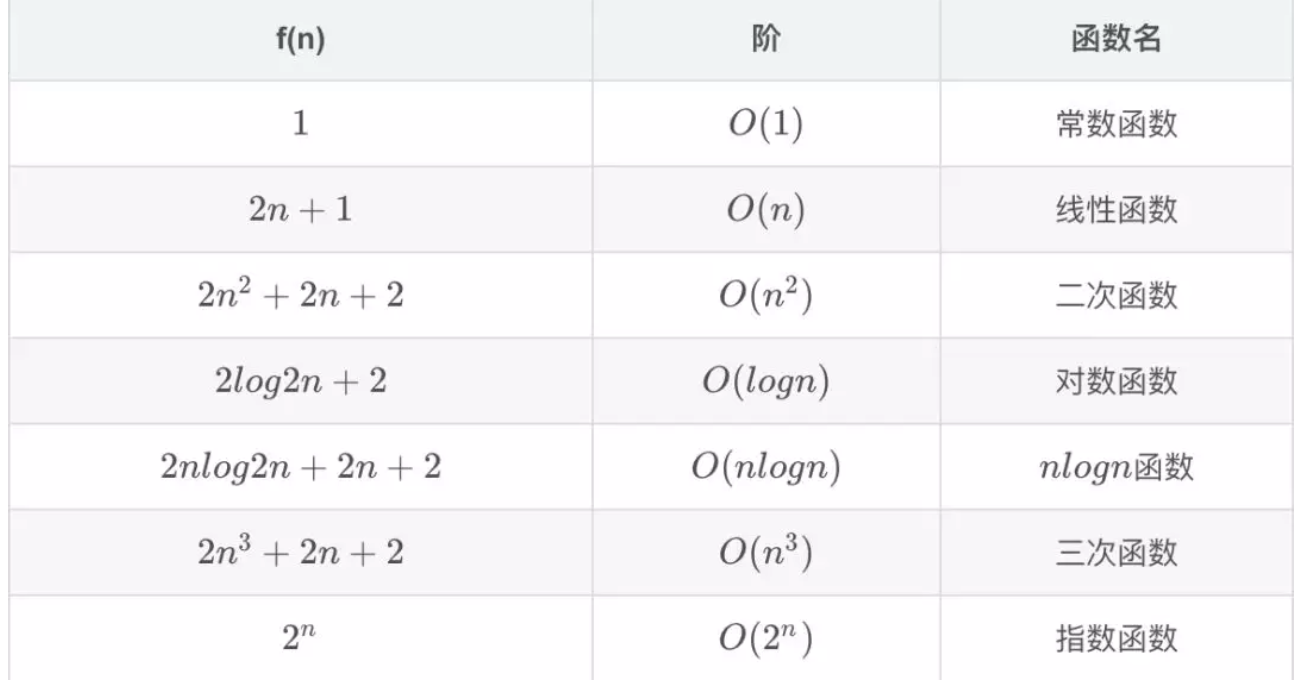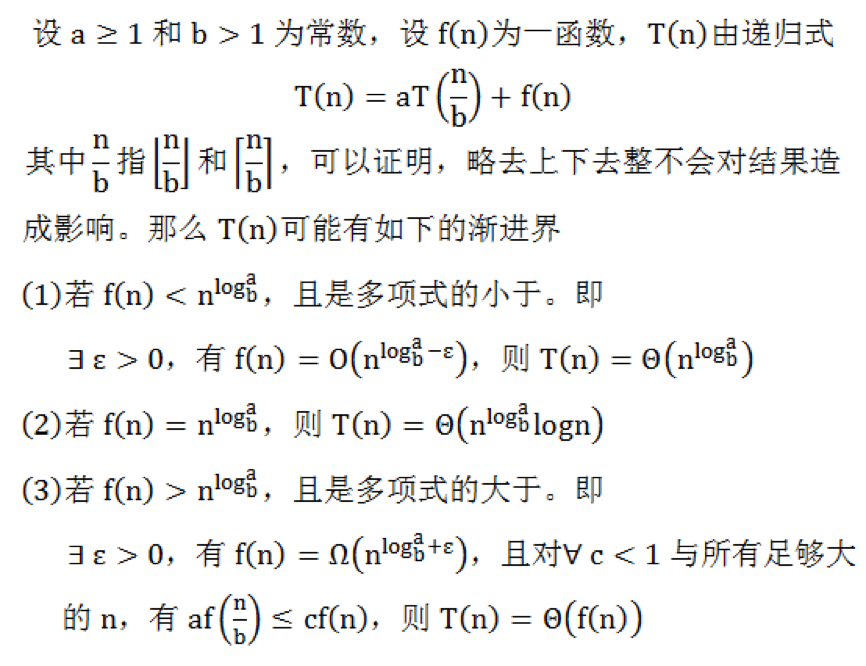一、浅谈算法

1.有穷性：不是数学，算法比较合理，每一步在规定时间内进行

2.确定性：每一条指令都有一个明确的含义

3.可行性：算法可以执行

4.输入0或者多个

5.输出 只有一个

1.正确性

2.可读性

3.健壮性：容错能力，输入数据非法的时候，不会产生的输出结果

4.效率和存储

2. 不关心编译语言，不关心机器

二、时间复杂度

1.概述

int sum=0;
for(int i=0;i<=n;i++){
sum=sum+i;
}

int i=0;
int sum=0;
sum =(1+n)*n/2;

2.时间复杂度的记法：

1.大O记号 （常用）

$当n>3的时候，2*n +2<3n，所以可选n0=3,c=3，则n>n0的时候，f(n)<c*(n)，所以f(n)=O(n)。$

2.Ω记号

$f(n) > c*g(n)$
Ω记号与大O记号相反，他可以表示算法运行时间的下界。$Ω（g（n））$表示的函数集合的函数是所有阶数超过g(n)的函数。

3.Θ记号

Θ记号介于大O记号和Ω记号之间。他表示，存在正常数c1,c2,n0，当n>n0的时候，$c1*g(n)≤f(n)≤c2*g(n)$，则f$(n)=Θ(g(n))$。他表示所有阶数与g(n)相同的函数集合。

4.小o记号

$f(n)=o(g(n))当且仅当f(n)=O(g(n))且f(n)≠Ω(g(n))$。也就是说小o记号可以表示时间复杂度的上界，但是一定不等于下界。

5.例子

f(n)=Ω(n^2)或者f(n)=Ω(n)或者f(n)=Ω(1)

f(n)=Θ(n^2)

f(n) = o(n^3)或者f(n)=o(n^4)或者f(n)=o(n^5)或者……

3.时间复杂度类型

1.常数阶

int i=0;
int sum=0;
sum =(1+n)*n/2;

2.线性阶

int sum=0;
for(int i=0;i<=n;i++){
sum=sum+i;
}

3.对数阶

while (left <= right) {
int mid = (left - right) / 2 + right;
if (target == nums[mid]) {
return mid;
} else if (target > nums[mid]) {
left = mid + 1;
} else {
right = mid - 1;
}
}

4.函数调用

main方法调用外部方法，两个方法都是一层循环，则$O(n^2)$

int main(int argc, char *argv[])
{
for(int i=0;i<n;i++){
fun(n);
}
}
void fun(int count){
for(int i=0;i<count;i++){
printf();
}
}

$O(1)<O(logn)<O(n)<O(nlogn)<O(n^2)...<O(n!)<O(n^n)$

4. 时间复杂度的计算

1.计算规则

1） 加法规则

$T(n,m) = T1(n) + T2(n) = O ( max (f(n), g(m) )$

2) 乘法规则

$T(n,m) = T1(n) * T2(m) = O (f(n) * g(m))$
$O(n)*O(m)=O(n*m)$

3)一个特例

1.用常数1取代所有的加法常数 t(n)=5 O(1)

2.修改后的函数中，只保留最高阶数

3.如果最高阶数的常数部分存在不是1，变成1。

$T(n) = n^3 + n^2 + 29，此时时间复杂度为 O(n^3)。 T(n) = 3n^3，此时时间复杂度为 O(n^3)$2.主定理void Hanoi(int n, char a, char b, char c)//a为原始柱，b为借助柱,c为目标柱
{
if (n == 1)
{
Move(a, c);//只有一个盘子时直接移
}
else
{
Hanoi(n - 1, a, c, b);//将A柱子上n-1个盘子借助C柱子移到B上
Move(a, c);//将A最后一个盘子移到C上
Hanoi(n - 1, b, a, c);//将B柱子借助空A柱子移到C上
}
}

三、空间复杂度

<script type="text/javascript" src="http://cdn.mathjax.org/mathjax/latest/MathJax.js?config=default"></script>
，MathJax可以解析Latex、MathML和ASCIIMathML的标记语言，就可以用\$ 解析了，使用就是正常的。

https://blog.csdn.net/qq_33274645/article/details/52688025

https://mp.weixin.qq.com/s/9njtnqfAatjmjPh4geETqAposted @ 2018-11-24 17:26 辰砂tj 阅读(...) 评论(...) 编辑 收藏